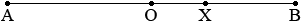SEARCH HOMEMath Central Quandaries & QueriesQuestion from rustom, a student: A point X is selected at random from a line segment AB with midpoint 0. Find the probability that the line segments AX, XB, and A0 can form a triangle. QuestionHi,

I drew a diagram with the point $X$ between $O$ and $B.$In order for three line segments to form the sides of a triangle it is necessary that the sum of the lengths of any two of the line segments be larger than the length of the third segment. Hence if $AX,$ $XB$ and $AO$ form the sides of a triangle you must have

$|AO| + |XB| > |AX|.$

But since $O$ is the midpoint of $AB$

$|AX| + |XB| = 2 |AO|.$

Solve this equation for $|AX|$ and substitute into the inequality above. What does this tell you about the placement of $X?$

Penny

Rustom wrote back

i don't get it, I arrived at AO + XB > 2AO - XB...but can't still figure out the probability thing?

Actually you should have $|AO| + |XB| > 2|AO| - |XB|$ where $|AO|$ is the length of the line segment $AO$ and so on. What this means is that you have an algebraic inequality and you can solve it. Subtract the number $|AO|$ from each side and add $|XB|$ to each side. But $|AO| = |OB|$ so you end up with

$2|XB| > |OB|.$

Which points $X$ in the interval $OB$ satisfy this inequality? Does the point $X$ in my diagram above? What about an $X$ close to $B?$ What fraction of the points $X$ between $O$ and $B$ satisfy the inequality? This is the fraction of the points between $O$ and $B$ for which $AX, XB$ and $AO$ can form a triangle.

PennyMath Central is supported by the University of Regina and The Pacific Institute for the Mathematical Sciences.